# Math – Basic math APK

•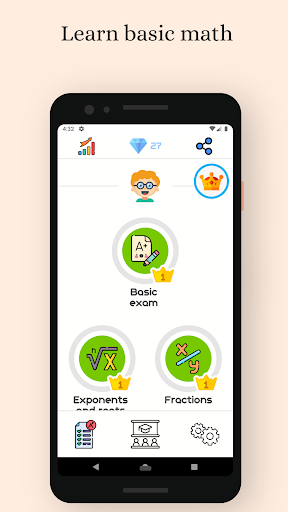•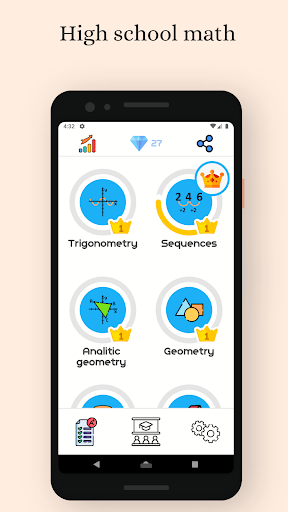•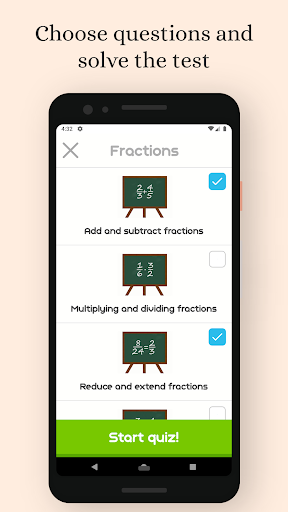•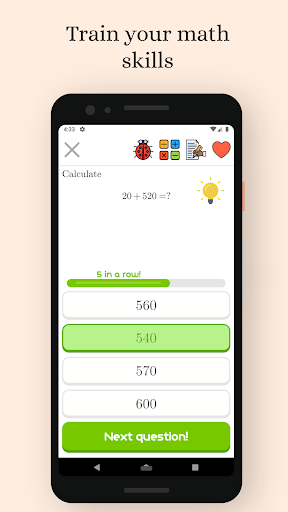•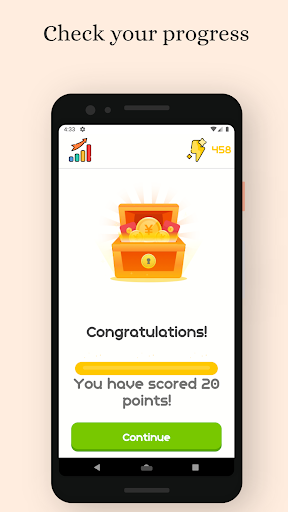•••••## App Information of Math - Basic math

 App Name Math - Basic math Category Apps, Education Developer MobileApps7 Package Name com.companyname.MaturaMatematyka Version 2.5 Rating 4 ( 25 ) Size 24.6 MB Requirement Android 5.1+ Updated 2020-10-02 Installs 5,000+

## Description of Math - Basic math

Basic Math :
App offers set of basic math tests and math practice questions for children, primary and secondary school students. You will receive a grade at the end of each math test. Use the application when you would like to learn basic math operations or speed up your calculation skills for your next competitive exam. If you are searching for a brain game to give your brain a workout, our application is for you!

Learn math :
Application is a great source of learning for children studying in kindergarten, primary or elementary School. There are some basic math questions like addition, subtraction, and multiplication, so app is a good choice for kids and primary school. Each question has an answer with explanation step by step. For high school students or college graduates, it can be a math practice tool to crack competitive exams or to improve math skills!

Math tests:
Each math topic has a lot of math questions. You can solve an easy or hard math test from every topic or you can prepare a math test for yourself by choosing the math practice questions form list. The app offers calculator and notes, so all you need for math learning is a telephone or tablet. You can learn for free and prepare for your math exams online!

The app consist of the following basic math problems:

– Roman numbers

– multiplication and division
– unit convertion
– order of operations

– percentages
– elements
– powers and roots
– fractions
– subtracting fractions
– decimal fractions
– algebra

For 6st,7st and 8st :
– equations
– system of equations
– basic geometry figures
– solid geometry

For secodary school students:
– logarithms
– short multiplication formulas
– theorem of sines and cosines
– sequences
– analytical geometry
– polynomials
Bug fix. New questions for classes 1-4